178simplify.html
Date: 06/12/05

## Analysis of Maths by Theosophical Reduction

It's a safe bet to give historic weight to the reduction of letter and number to single digits, if one wishes to see the simple, natural patterns of words and of long strings of numbers.

An example of this reduction of letters, is in one case, based upon writing out all of the 26 letters of the modern alphabet and traditionally assigning a sequential number to each letter, beginning with 1 for the letter A. This is called Theosophical reduction......namely A=1, B=2, C=3 etc.

Once letters are converted to number, there is no logical reason that any string of numbers cannot be further reduced to single digits:

### Number 9 Only

Note: The numeric equivalent of the word DOUBLE LIGHT is 52=7
but gematric 288 is also double the gematric 144 traditionally assigned to light.

Double Light= 288 = 9
Double Light Squared = 82944 = 9
82944 Squared = 68979707136 = 9

### Numbers 1-8

Whenever this technique is applied to long strings of numbers, it reveals that all number, no matter what the size of the string, ignoring the placement of the decimal, IS EQUIVALENT (=) to a single number from 1-9 and no more numbers are needed because 10= 1+0= 1 again, and so on repeating singly, forever.

### Pertaining to All Number:

Multiplication of: 1...1x1=1...1x1x1=1.....................Series: 1,1,1

Multiplication of: 2...2x2=4...2x2x2=8.....................Series: 2,4,8

Multiplication of: 3...3x3=9...3x3x3=9.....................Series: 3,9,9

Multiplication of: 4...4x4=7...4x4x4=1.....................Series: 4,7,1

Multiplication of: 5...5x5=7...5x5x5=8.....................Series: 5,7,8

Multiplication of: 6...6x6=9...6x6x6=9.....................Series: 6,9,9

Multiplication of: 7...7x7=4...7x7x7=1.....................Series: 7,4,1

Multiplication of: 8...8x8=1...8x8x8=8.....................Series: 8,1,8

Multiplication of: 9...9x9=9...9x9x9=9.....................Series: 9,9,9

### Pertaining to All Number:

In Addition the First Column progresses: 1,2,3,4,5,6,7,8,9
*Notice this is exactly like the series of First Column in Multiplication.

In Addition the Second Column progresses: 2,4,6,8,1,3,5,7,9
**This should have a relationship with Second Column in Multiplication.

In Addition the Third Column progresses: 3,6,9,3,6,9,3,6,9
***Notice the traditional enneagram "gap" 3,6,9 series is similar to 1,8,9
with 3 and 6 (0ctave?) alternating rather than 1 and 8 alternating.

In Multiplication the First Column progresses: 1,2,3,4,5,6,7,8,9
*Notice this is exactly like the series of First Column in Addition.

In Multiplication the Second Column progresses: 1,4,9,7,7,9,4,1,9
**This should have a relationship with Second Column in Addition.

In Multiplication the Third Column progresses: 1,8,9,1,8,9,1,8,9
***Notice the "new gap" 1,8,9 series is similar to Rodin's 3,6,9
with 1 and 8 (0ctave) alternating rather than 3 and 6 alternating.

### Pertaining to Relationships Between Addition and Multiplication:

Notes: So far with number reduced to one digit, there arises eternal patterns in all relationships between addition and multiplication:

• *The series of the First Columns of both Addition and Multiplication are exactly the same. Numbers 1,2,3,4,5,6,7,8,9.
• **The Addition of the Second Column has a relationship with Multiplication of the Second Column. 2,4,6,8,1,3,5,7,9 : : 1,4,9,7,7,9,4,1,9
• ***The Addition of the Third Column has a relative "gap" as does the Multiplication of the Third Column. 3,6,9,3,6,9,3,6,9 : : 1,8,9,1,8,9,1,8,9

From this organization and reduction of all number, we can make certain informed deductions, based upon a certain premise that:

The basis of all matter is toroidal, therefore all number can be properly mapped upon a toroidal form, which will then describe the function of all matter.

To demonstrate why this should occur, let's intelligently look at certain parts of our above conclusions to see if we can tie toroidal forms with number.

* The similarity of the series of numbers in the First Column of the Addition and Multiplication tells me that it is the basis and common origin of counting systems devised by Man. Universe only understands 0 (zero) and one 0 (zero), however, if we wish to demonstrate any razzle-dazzle, someone had to invent the number 1 as a counting unit so we can add 1 to 0, so we can begin to count anything. This was a total invention and a completely artificial move but very necessary to modern mathematics. Previous studies note that all numbers can be reduced to only 1-9 and can be lined up under the digits 0 and 1, only, as resonant conditions. When you think of this series, try to imagine resonant conditions between the "real" numbers and 0,1:

0,1
2,3
4,5
6,7
8,9

We can also add another -1 so that the pair (0,1) turns into the triplet (-1,0,1), the yin/yang -/+ with neutral 0 in the middle:

-1,0,1
2,0,3
4,0,5
6,0,7
8,0,9

** How are we going to interpret the diverse series of numbers of the Second Column of both Addition and Multiplication?

2,4,6,8,1,3,5,7,9 : : 1,4,9,7,7,9,4,1,9

Let's treat both as ratio. The : : sign means "as is". This means series 2,4,6,8,1,3,5,7,9 is to itself "as is" 1,4,9,7,7,9,1,4,9 but I see a pattern within each one, so let's split both up like they should be split, by moving the 9(0) to the center:

2,4,6,8.........9(0).......1,3,5,7
OR
1,4,9,7..........9(0).......7,9,1,4

The split makes it obvious that the reality of so-called "Real" Numbers has two sides to be considered. A "center" must also be considered. One side seems to have 4 of something and the other side also has 4 of something with a extra 9 center for each side. We can see this asymmetry is just like B. Fullers "bowtie 9". This bowtie can be plotted around the surface of the torus. Just imagine one mobius loop making one circuit. These juxtaposed numbers may be equal and cancel or they may be ambidextrous, inverted, reciprocal, complimentary, unequal partners. Only the intelligent will know the details, which I have collected on this site, herein.

*** The 3,6,9 "gap" of previous study, tells me that the 9 is 0-center of certain enneagram number systems and the 3 and 6 are oscillations just as B. Fullers 9(0) center is the center of the oscillating +/- 1 to 4. We also discover here that the triplet 3,6,9 has a triplet partner 1,8,9 which also acts like B. Fuller's system, and by intuition, works inverse from 3,6,9, both upon the surface of the torus. We might go further and say that whenever you see the single digit 1,3,6,8 or 9(0), you may conclude that you are seeing a (9) center with two pair of forces juxtaposed against each other:

1 against 3, 6 against 8
1 against 6, 3 against 8
1 against 8, 3 against 6
etc.
In how many combinations?

4x4 = 16 Didactic Functions

So, if we want to abandon our simple number 288 and complicate our formulae by squaring 288 to equal 82944 and then square that (82944^2 = 6879707136), we should then square all of the other numbers along with 82944^2, then we will maintain all of the formulae and relationships. We can also apply Theosophical reduction to all these long numbers and formulae and reduce all of it to one-digit numbers.

Example: 6879707136= 6+8+7+9+7+0+7+1+3+6= 9 just as 82944 was reduced to 9.

If 82944 is valid, in the first complicated Iuliano formulae, then the reduction of 82944 to 9, a simple single-digit formulae, is also valid. Likewise, if Morton's formulae is reduced to single digits, it is also valid, although one cannot easily understand what 9 / 4 = 8 means, by way of a multiplication.

Although these simple insights may seem like child's play to others, more advanced, I would choose to take complicated maths and reduce them to simplicity.

### Theosophical Reduction and Jerry Iuliano's Complicated Maths on the Angular Measuring System: Degrees / Radian

From: Jerryiuliano@aol.com
To: rgrace@rgrace.org
Date: 03/23/04

MP:

From the unification equation, uniting two angular measuring systems..DEGREES...Sumerian ( 360 )..and RADIAN...1/2 Sumerian divided by Pi..180 / Pi = 57.29577951..Ę cosine = cos ...radians and COSINE = COS...degrees:

Letting fine-structure constant = 1/137.036004346 = aem
...then it can be demonstrated: i = sqrt( - 1 )
(( cos 137.036004346 ) ^ ( COS 137.036004346 )) / 10 = i ^ i = 1 / sqrt ( e^Pi )
...solving for exponential x using master harmonic 82944 in this form:
82944 ^ x = COS 137.036004346...degrees
82944 ^ -.027571485 = COS 137.036004346...degrees
...which decays (natural log e ) to the electron in million electron volts:
(( e ^ ( e ^ ( e ^ -.027571485 ))) / 10 ) / cos 137.036004346 = 1/ ( emev ^ 2 )
...where emev = .5109981152 mev....( 2002 NIST = .510998918 mev )
The master harmonic 82944 is equivalent through the electron form:
((( e ^ ( e ^ ( e ^ -.027571485 ))) * 37 * 17.9995953 ) ^ ( emev ^ ( - 1/Pi )) = 82944
...where emev = exact 2002 NIST valueĘ=Ę.510998918... mev

J.Iuliano

### The Simple Version of Jerry Iuliano's Maths on the Angular Measuring System: Degrees / Radian

From: Jerryiuliano@aol.com
To: rgrace@rgrace.org
Date: 03/23/04

MP:

From the unification equation, uniting two angular measuring systems..DEGREES...Sumerian ( 9 )..and RADIAN...1/2 Sumerian divided by Pi.......9 / Pi = 3..Ę cosine = cos ...radians and COSINE = COS...degrees:
Letting fine-structure constant = 1/1 = aem
...then it can be demonstrated: i = sqrt( - 1 )
(( cos 1 ) ^ ( COS 1 )) / 1 = i ^ i = 1 / sqrt ( e^Pi )
...solving for exponential x using master harmonic 9 in this form:
9 ^ x = COS 1...degrees
9 ^ -.3 = COS 1...degrees
...which decays (natural log e ) to the electron in million electron volts:
(( e ^ ( e ^ ( e ^ -.3 ))) / 1 ) / cos 1 = 1/ ( emev ^ 2 )
...where emev = .5 mev....( 2002 NIST = .5 mev )
The master harmonic 9 is equivalent through the electron form:
((( e ^ ( e ^ ( e ^ -.3 ))) * 1 * 4 ) ^ ( emev ^ ( - 1/Pi )) = 9
...where emev = exact 2002 NIST value = .5... mev

J.Iuliano

How do we read such single digit numbers?

### Theosophical Reduction and Michael Morton'sComplicated Maths on: Stellar Proof....Corrected

Note: This is only a small section of the email.

99.5.2 Stellar Proof....Corrected

SOLAR APEX Grid LAT .. 53 (deg) X 26 (min) X 27.00111177 (sec) North of ecliptic .. = 37207.53202 North of ecliptic.

SOLAR APEX Grid LONG .. 173 (deg) X 13 (min) X 47.02552383 (sec) W.ALNITAK .. = 105760.4031 W.ALNITAK.

SOLAR APEX Grid POINT Value .. 105760.4031 / 37207.53202 = 2.842446068

### The Simple Version of Michael Morton's Maths on: Stellar Proof....Corrected

SOLAR APEX Grid LAT .. 8 (deg) X 8 (min) X 9 (sec) North of ecliptic .. = 4 North of ecliptic.

SOLAR APEX Grid LONG .. 2 (deg) X 4 (min) X 3 (sec) W.ALNITAK .. = 9 W.ALNITAK.

SOLAR APEX Grid POINT Value .. 9 / 4 = 8

How do we read such single digit numbers?

### Theosophical Reduction and the Toroidal Number Series 3,6 and 9.

We'll begin with the proper image: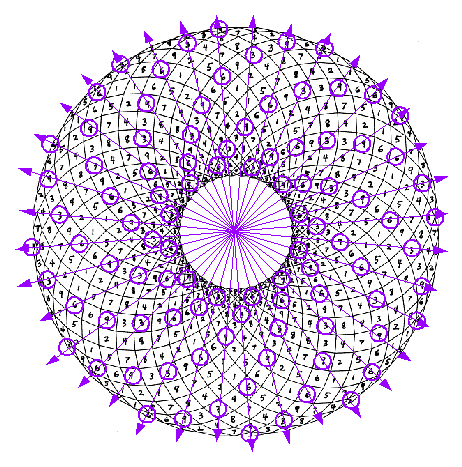Courtesy of M.Rodin

In this counterwound coil there is a 3,6,9 series but the series goes like this:

3,3,9,6,6,9,3,3,9,6,6,9

The author of this winding scheme, doubles each oscillating 3 and 6. Is this Reality and necessary or an error? Notice that each 9 is surrounded by eight other numbers. Each two of these numbers directly across the 9, from each other, all add to 9. Because of the repeated 3,3's and 6,6's the numbers surrounding these repeats, do not add to 9, yet the straight, radial lines go right through other 3,6 and 9's making up every spiral. Are these spirals, "the prime spirals"?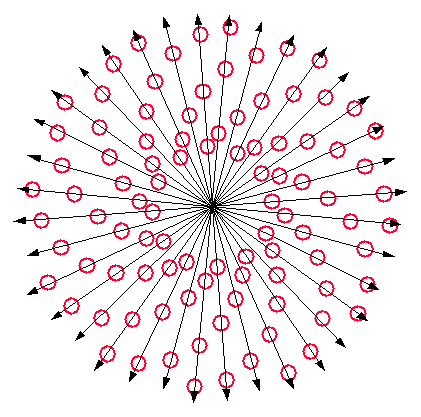Courtesy of M.Rodin

This radial/spiral view, also has the 3,6,9 series but the series, again, goes like this:

3,3,9,6,6,9,3,3,9,6,6,9

Here we can discern how spirals originate.

Each circle is either a 3,6 or 9 according to Rodin.

However in previous study we found that, after Theosophical Reduction of numbers to single digits, any single number that is 3,6 or 9 is not a prime. If any single digit is 1,2,4,5,7 or 8, it is a prime. (P.Plictha) Since these are 3,6 or 9, they must not be primes.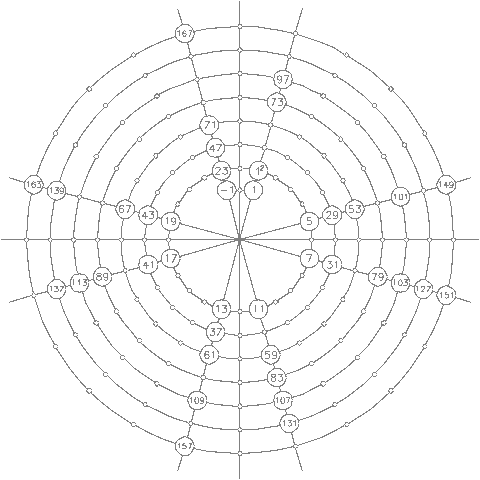Courtesy of P.Plictha

The rationale for this is rooted in Peter Plictha's Prime Number Cross. If we reduce any number to a single digit we find what Plictha found. That is: 6 and all multiples of 6 cannot be primes because all number on each side of 6 and multiples of 6 are the primes. We can also note that 6's and all multiples of 6, always reduces to 3,6 or 9 so any 3,6 or 9 is never prime. For example:

6=6
12=3
18=9
24=6
30=3
36=9

### Theosophical Reduction. What did we discover?

In this file, "Analysis of Maths by Theosophical Reduction" we discovered that the center "gap" series was 3,6,9,3,6,9 (Assume no primes) with a partner "gap" series 1,8,9,1,8,9 (Assume primes)

In this file we also discovered that each series to each "side" of the center 9(0) was:

2,4,6,8.........9(0).......1,3,5,7
OR
1,4,9,7..........9(0).......7,9,1,4

Tony Smith names these two juxtaposed series as,

"1,2,4,8,7,5... and another doubling sequence going in the opposite direction 5,7,8,4,2,1...."

### What did we discover previously about Partner Numbers?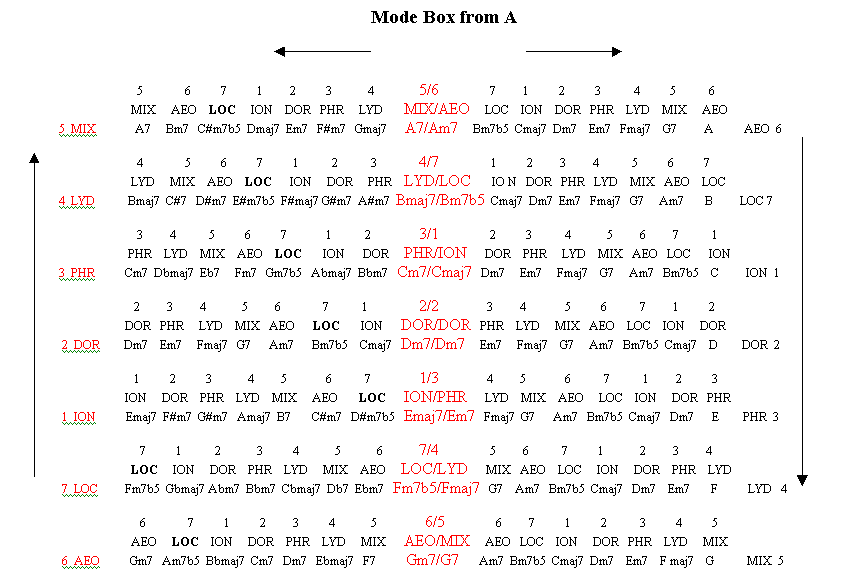Courtesy of L.Di-Martino

There are certain Ancient Greek scales that are 6 double octave sets surrounding a center, 7th double set called the Dorian/Dorian center pair, or in total, 13 double sets, which demonstrates sets against sets in a complimentary manner of pairings. One-half of each set of octaves also seemed to "travel" backward.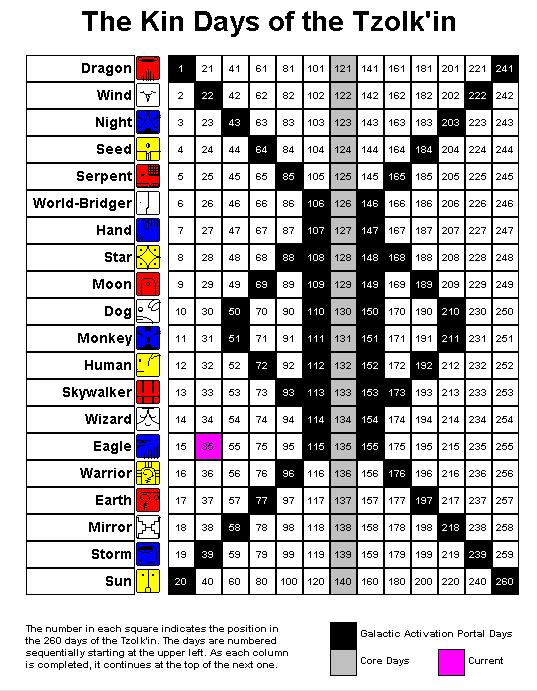Courtesy of L.Di-Martino

In the Mayan Tzolkin, one of the main characteristics of its numberings, is that the very last kua (number) is a complimentary partner of the very first kua (number). This complimenting holds for every other numbered pair of the entire 260 kua. One-complimentary-half of each set of kua also seems to "travel" backward. No matter what direction the kua seem to "move", they are always in pairs. This is the Template of Light.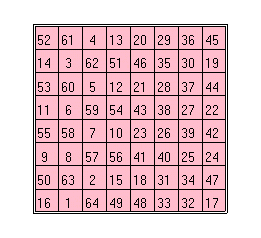8x8 I-Ching as Magic Square of B.Franklin

In the Chinese I-Ching, there are also 32 of 64 numbers that are also perfectly positioned so as to be complimentary to its other perfectly positioned partners, directly across the center. One-complimentary-half of each set of numbers also seemed to "travel" backward. One-complimentary-half of life, which is governed by the I-Ching, also seems to be "traveling" backward. One-complimentary-half of DNA, which is governed by this I-Ching, also seems to be "traveling" backward. No matter what direction the kua seem to "move", they are always in pairs and also always add to 260. This is the Template of Life.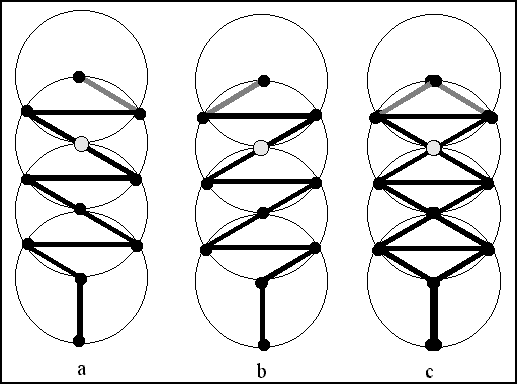Double Sepiroth

The Sepiroth of Kaballic mysticism even seems like it should be doubled.

### Commentary On Theosophical Reduction by Derek Skhane

Note: My Theosophical reduction of numbers are in (parentheses).
Derek's Theosophical reduction of numbers are in {brackets}.
I began with 2 and 3 digit numbers so the larger numbers will eventually contain the 2 and 3 digit sums.
A larger number which has (parentheses) within it, such as 370(1)370(1)370(1)(3), can still be read as 370370370(3), as the (1),(1),(1) adds to (3).
Most numbers sum to 3,6 or 9 and seem most harmonic.

Robert

As you are aware, the number 9 possesses mathematical properties galore, I am uncertain whether this snippet will be of assist, nevertheless here it is

Your number sequence: 111(3), 248(5), 399(3), 471(3), 578(2), 699(6), 741(3), 818(8), 999(9) adds to: 5064(6) if we deduct 3024(9) {Great Pyramid} from 5064(6) we obtain 2040(6) and this x 0.9 = 1836(9) the roofline length {sloping} of the Grand Gallery

The number Addition sequence you illustrate for the Second Column being:

2, 4, 6, 8, 1, 3, 5, 7, 9, likewise the Multiplication sequence of the Second Column

1, 4, 9, 7, 7, 9, 4, 1, 9

Then: 246(3) + 813(3) + 579(3) = 1638(9) a rearranged 1836(9) therefore 1836(9) minus 1638(9) = 198(9) and this x 15.272727(6) = 3024(9) the 15.272727(6) x 33(6) = 504(9) Plato's Magnesia number, likewise your Multiplication in the Third Column shows: 1, 8, 9, 1, 8, 9, 1, 8, 9

Likewise: 149(5) + 779(5) + 419(5) = 1347(6) this equates as: 13(4) + 47(2) = 60(6) this deducted from the 5064(6) = 5004(9) which is the product of your series: 123(6), 246(3), 369(9), 483(6), 516(3), 639(9), 753(6), 876(3), 999(9).

Your number sequence of the Third Column shows: 3, 6, 9, 3, 6, 9, 3, 6, 9

Then 369(9)369(9)369(9) divided by 189189189(9) = 1.952380952380(1) and this x 63 = 123(6)

Then the penultimate number 818(8) divided by the first number 111(3) = 7.369(9)369(9) there is the 3, 6, 9 sequence

The 7.369(9)369(9) x 999(9) = 7362(9) this minus 3024(9) minus 1836(9) minus 504(9) = 1998(9) {3 x 666(9)} It simply endures perpetually Robert, therefore I shall finish at this point

Derek Skhane
Reflective Math

________________________________

Robert

The following may assist regarding the ongoing "What's needed to make this work?"

Mathematical Model

Number is the overriding connective influence to every field of research whether it is geodesy, astronomy, metrology or a host of other types. Let us observe what ensues commencing with the fundamentals, heeding the fact that the number 9 {nine} is the fundamental of all number

The objective in the following exercise is to add the three numbers together then deduct them from the object number, for example 111(3) adds up to 3 and 111(3) minus 3 = 108(9), then 1 + 0 + 8 = 9

111(3) minus 3 = 108(9) {1 + 0 + 8 = 9} the 108(9)9(9) + 108(9)0(9) = 216(9)9

222(6) minus 6 = 216(9) {2 + 1 + 6 = 9} the 216(9)9 + 108(9)0 = 3249

333(9) minus 9 = 324(9) {3 + 2 + 4 = 9} the 3249(9) + 108(9)0 = 432(9)9(9) etc, etc. Note the downward emergence of 234.123(6) in the right hand column likewise the 642(3) and the 999(9) given that 999(9) minus 642(3) = 357(6) and this minus 123(6) = 234(9)

Alternatively

444(3) minus 12 = 432(9) {4 + 3 + 2 = 9} the 432(9)9(9) divided by 108(9)0(9) = 4.008333(9)3(3)33(3) which x 5409(9) = 216(9)81.075, this x the FP = 2284(5)0.96790123(6)456790123(6)45 which divided by 1.185185(5) {music: 32:27} = 19272.0666(9)66(6) and this divided by 648 then multiplied by 108(9)0 = 32120.111(3)111(3)11 which divided by the 216(9)81.075 = 1.481481(9) which x 243(9) = 360(9) degrees {108(9)0 is the lunar number likewise 108(9)0 x 2.8 = 3024(9) Great Pyramid. Likewise 108(9)0 is 864(9) + 216(9), etc}

555(6) minus 15 = 540 {5 + 4 + 0 = 9}

666(9) minus 18 = 648 {6 + 4 + 8 = 18}

777(3) minus 21 = 756(9) {7 + 5 + 6 = 18} the 756(9) = Great Pyramid

888(6) minus 24 = 864(9) {8 + 6 + 4 = 18} the 864(9) is the 7th term of PWS {Plato's World Series}

999(9) minus 27 = 972(9) {9 + 7 + 2 = 18} the 972(9) is the 8th term of PWS {Plato's World Series}

101010(3) minus 3 = 101007 {1 + 0 + 1 + 0 + 0 + 7 = 9}

111(3)111(3) minus 6 = 111(3)105 {1 + 1 + 1 + 1 + 0 + 5 = 9}

There we possess the basis for a whole host of additional numbers

Whilst the three digit numbers are increased to four digits, following the same modus operandi multiples of 9 will forever arise for example

111(3)1 minus 4 = 1107 {1 + 1 + 0 + 7 = 9}

222(6)2 minus 8 = 2214 {2 + 2 + 1 + 4 = 9}

333(9)3(3) minus 12 = 3321 {3 + 3 + 2 + 1 = 9}

444(3)4 minus 16 = 4428 {4 + 4 + 2 + 8 = 18}

555(6)5 minus 20 = 5535 {5 + 5 + 3 + 5 = 18}

666(9)6 minus 24 = 6642 {6 + 6 + 4 + 2 = 18}

777(3)7 minus 28 = 774(9)9 {7 + 7 + 4 + 9 = 27}

888(6)8 minus 32 = 8856 {8 + 8 + 5 + 6 = 27}

999(9)9 minus 36 = 9963(9) {9 + 9 + 6 + 3 = 27}

101010(3)10 minus 4 = 101010(3)06 {1 + 0 + 1 + 0 + 1 + 0 + 0 + 6 = 9}

111(3)111(3)11 minus 8 = 111(3)111(3)03 {1 + 1 + 1 + 1 + 1 + 1 + 0 + 3 = 9}

Likewise again note the two downward patterns of 123(6)456789 {there are supplementary patterns within}

If we utilize the 999(9) product 864(9), employing the same methodology the following occurs

864(9) minus 18 = 846(9) minus 18 = 828(9) minus 18 = 810(9) minus 9 = 801(9) minus 9 = 792(9) minus 18 = 774(9) minus 18 = 756(9) {Great Pyramid} etcetera, plus the following obviously ensues

111(3) + 222(6) + 333(9) + 444(3) + 555(6) + 666(9) + 777(3) + 888(6) + 999(9) = 4995(9) then reverse the 4995 to 5994(9) then add both to produce 10989(9) which minus 4374(9) = 6615(9) which minus 54 = 6561(9)

This occurs via: 10989(9) minus 9963(9) = 1026(9) which + 3348(9) = 4374(9), the 3348(9) minus 18 = 333(9)0 which is obviously 30 x 111(3)

4374(9) is the 25th term of PWS {Plato's World Series}

6561(9) is the 30th term of PWS {Plato's World Series}

Derek Skhane
Reflective Math

________________________________

Robert

Continuing on, your number sequence: 246(3)813(3)579(3) add to this 123(6)456789 to obtain: 370(1)270(9)368(9) {this plus 100002(3) = 370(1)370(1)370(1)(3)}

Then: 370(1) x 270(9) x 368(8) = 36763200 and: 36 + 763 + 200 = 999(9)

Alternatively: 370(1) + 270(9) + 368(8) = 1008(9) {twice 504(9)} a pair of amicable numbers acknowledged by the Pythagoreans being 284(5) and 220(4) hence: 284(5) + 220(4) = 504(9), the proper divisors of 220(4) are 1, 2 , 4, 5, 10, 11, 20, 22, 44, 55 and 110 they add up to 284(5). The proper divisors of 284(5) are: 1, 2, 4, 71 and 142 they add to 220(4)

Your 246(3)813(3)579(3) minus 123(6)456789 = 123(6)356790 this + 999(9)99 = 123(6)456789

There is a need to add in wherever the numbers 10, 9, and 1

Therefore we utilize them as 1091(2), the numbers 8712(9) and 9801(9) are the only four-digit numbers that are integral multiples of their reversals {G.H.Hardy: Mathematicians Apology}

108(9)9

2178(9)

3267(9)

4356(9)

5445(9)

6534(9)

7623(9)

8712(9)

9801(9)

Then 9801(9) minus 1091(2) = 8710(7) + 0178(7) = 888(6)8 + 111(3)1 = 999(9)9

Then 1091(2) minus 108(9)9 = 2 deduct 2 from the above 0178(7) to obtain 176(5)

Then: 123(6)456789 + 370(1)370(1)369(9) = 493827158(2) ( that minus 246(3)813(3)579(3) = 247013579

Then: 247013579(2) ( minus 200000 = 246(3)813(3)579(3)

To regain the previous email total of 5064(6), then: 1091(2) + 1901(2) = 2992(4) from this deduct your 111(3) then your 818(8) to obtain: 2063(2) + 3001(4) = 5064(6)

At commencement I stated: Continuing on, your number sequence: 246(3)813(3)579(3) add to this 123(6)456789 to obtain: 370(1)270(9)368(8)

Then: 370(1)270(9)368(8) plus 100002 = 370(1)370(1)370(1)(3), the 100002 plus 999(9)9 = 110001(3) + 111(3)0 = 111(3)111(3)

Then: 370(1)370(1)370(1)(3) minus your number sequence: 369(9)369(9)369(9) = 1001001(3) that plus 110110(4) = 111(3)111(3)1

The Great Pyramid unit of 432(9)432(9) {756(9) x 572} divided by the above 110110(4) = 3.9272727(3) that x 220(4) = 864(9) or 3.9272727(3) x 660(3) = 2592(9) etc, etc

Likewise the 370(1)370(1)370(1)(3) minus your 189189189(9) = 181181181(3) which is 181(1) x 1001001(3) {above} seeing as a rearranged Grand Gallery unit {1881(9)} is within the 181181181(3), the following is worth a mention

All cyclic numbers are the periods in the repeating decimals of the reciprocals of certain prime numbers, the reciprocal of 1/7 is the repeating decimal 142857(9) {note there are 6 repeating numbers not 7}.

Now one divided by seven produces: 0.142857(9)142857(9) and this x 1267(7) = 181 {above} the 1267(7) the 12 + 67 = 79 + 97 = 176(5)

Alternatively: 1267(7) minus 111(3)1 = 156 this is half of the Maya Third Sun {312} likewise 156 x 3.666(9)666(9)6 = 572 the capstone, likewise 156 x 876(3)0 = 1366560 the Maya "super" number. The 876(3)0 minus your total of 5064(6) = 369(9)6 that minus your 369(9)3 = 3

The next larger prime that generates such a number as discussed above is 17 {17 x 108(9) = 1836(9) the Grand Gallery} likewise the addition of one through to seventeen obtains 153(9) and 153(9) x 12 = 1836(9). Therefore one divided by seventeen = 0.0588(3)235(1)294(6)117(9)6(5)47, {note there are 16 repeating numbers not 17}

Then: 588(3) + 235(1) + 294(6) + 117(9) + 647 = 1881 the Grand Gallery unit

Likewise within the digits, clearly seen is 882, 294(6), 117(9)6(5) and 176(5)4 all full of symbolism in this instance we utilize 117(9)6(5) {Only/begotten Son} {117(9)6(5) is 1.333(9)3(3)33 x 882 = City of God, and 117(9)6(5) is 4 x 294(6) = Church}

Hence: 117(9)6(5) divided by 0.0588(3)235(1)294(6)117(9)6(5)47, = 1999(9)2 + 8 = 20000, otherwise 1999(9)2 minus 117(9)6(5) minus 117(9)6(5) = 176(5)40 = 60 x 294(6) or else 176(5)40 = 20 x 882, etc, etc

The number 37 pertains to the "Guardians" of the Law in Plato's Laws V1 and 37 x 27 = 999(9)

I trust this is of a little assist, as the combinations within Robert, are literally numerous

Derek Skhane
Reflective Math

### Conclusions

By Theosophical Reduction most numbers reduce to 3's and 9's with 9's leading. The arbitrary numbering schemes of logic, applied to number and toroids, is often different between one author and another. However it is certain that there is a neutral oscillation (+/0/-) between two opposite directions of information flow. Theosophical Reduction removes the arbitrary number schemes so that proper, Theosophically reduced, single-digit number schemes can be applied to the toroid, for the desired results.

Impossible Correspondence Index